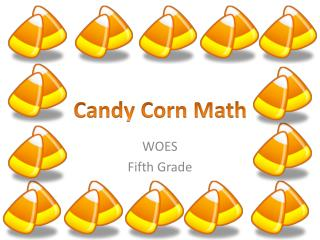DownloadDownload Presentation# WOES Fifth Grade

Download Presentation## WOES Fifth Grade

- - - - - - - - - - - - - - - - - - - - - - - - - - - E N D - - - - - - - - - - - - - - - - - - - - - - - - - - -
##### Presentation Transcript

1. Candy Corn Math WOES Fifth Grade

2. Benchmarks • A benchmark is a familiar number used as a point of reference. You can use a benchmark to determine a reasonable estimate. • If one piece of candy corn is: • Remember your base 10 rules: n x 1= 1, n x 10= 10, n x 100= 100 Height: in. cm. Weight: oz. kg. Length: in. cm.

3. Measurement Charts Length Standard System METRIC System

4. Reading a Ruler • Standard: http://www.rsinnovative.com/rulergame/ • Metric: http://www.askmrcscience.net/sitebuildercontent/sitebuilderfiles/readingametricruler.pdf

5. Station One: Length You will need a ruler that measures standard and metric systems. • How many centimeters long is 1 candy corn? • How many cm. long are 10 candy corns? • How many cm. long is 100 candy corns? • How many candy corns are needed to reach 6 in.? • Estimate the length of all of your candy corns in cm. and in inches. • What is the actual length of all of your candy corns in cm. and in in.?

6. Measurement Charts Weight/ Mass StandardSystem METRIC System 1 milligram = 0.001 gram1 centigram = 0.01 gram1 decigram = 0.1 gram1 kilogram = 1000 grams

7. Station Two: Weight/Mass You will need a scale and weights that measures using the metric system. • What is the weight of 10 candy corns in grams? • How many candy corns would equal 1 kilogram? • If each candy corn costs \$0.02, what would be the cost of one kilogram? • If each candy corn costs \$0.02, what would be the cost of 200 grams?

8. Measurement Charts Capacity 1 cup (c.)= 8 fluid ounces (fl. oz.) 1 pint (pt.)= 2 cups (c.) 1 quart (qt.)= 4 cups (c.) 1 quart (qt.)= 2 pints (pt.) 1 gallon (gal.)= 4 quarts (qt.) Liquid Dry 1-1/2 tsp.= 1/2 tbsp. 3 tsp. = 1 tbsp. 2 tbsp. =1/8 cup 4 tbsp. =1/4 cup 8 tbsp. =1/2 cup 16 tbsp. = 1 cup

9. Station Three: Capacity You will need a tsp., tbsp., measuring cup and a pint jar. Estimate first then check. • How many candy corns will fit in a teaspoon? • How many candy corns will fit in a tablespoon? • How many candy corns will fit into a cup? • How many candy corns will fit into a pint? 5. How many candy corns will fit into a quart? 6. How many candy corns will fit into a gallon? Now, use your Math knowledge :

10. Finding Range, Mean, Median and Mode Range refers to the distance “Between”. The Rangerefers to the distance between the highest and lowest value within a set of numbers. Strategy: Order your data from greatest to least. Mean means “Average”.Mean = the average of a group of numbers. Strategy: Add all numbers then divide by the number of entries. Medianmeans is in the “Middle”. Strategy: Order the numbers from least to greatest. Mode represents the most “Popular”The Mode is the number that appears the most frequently within a set of number. Strategy: What number do you see the most often?

11. Station Four: Finding Range, Mean, Median and Mode • Time each member of your group to find out how long it takes to eat one candy corn. DONOT RUSH! Record your answers in minutes and seconds. • Find the range of the times. • Find the median of the times. • Find the mode of the times, if there is one. • Find the mean of the times. • Make a stem and leaf plot to represent your data. You will need a stopwatch.

12. Fractions: Review The top number the Numerator, it is the number of parts you have. The bottom number the Denominator, it is the number of parts the whole is divided into. Hint: Denominator- “down”-ominator

13. Station Five: Fractions • Divide the candy corns into two equal groups. What is ½ of the total number? • Divide the candy corn into four groups. How many are in each: • How many are in 2/4 ? • How many are in ¾ ? • How many are in 4/4 ? • Divide the candy corns into sixths. a. What does 1/6 equal? b. What does 2/6 equal? c. What does 3/6 equal? d. What does 4/6 equal? e. What does 5/6 equal? f. What does 6/6 equal? 4. What fractional parts had the same number of candy corns?

14. Station Six: Word problems Try to write each problem using a variable. 1. addition 2. subtraction 3. multiplication 4. division 5. inequality Try to solve each other’s problems.